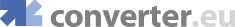online flow conversions, online flow mass conversions, gram/second, kilogram/hour, pound/hour
Engineering converters
Heat converters
Fluids converters
 Concentr. Liquid Solution Concentration - Molar Flow Flow - Mass Flow - Molar Mass Flux Density Permeability Surface Tension Viscosity - Dynamic Viscosity - Kinematic
Light converters
Electricity converters
Magnetism converters
Other converters

 Show currency exchange rate: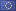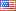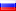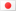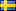… Get a Converter API tool for your website for free!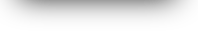All Flow - Mass Conversion
Convert fromConvert to
g/s =  g/s
 Common units Gram/Second g/s Kilogram/Hour kg/h Pound/Hour lb/h Other units Centigram/Second cg/s Decigram/Second dg/s Dekagram/Second dag/s Exagram/Second Eg/s Gigagram/Second Gg/s Gram/Day g/day Gram/Hour g/h Gram/Minute g/min Hectogram/Second Hg/s Kilogram/Day kg/day Kilogram/Minute kg/min Kilogram/Second kg/s Megagram/Second Mg/s Microgram/Second µg/s Milligram/Day mg/day Milligram/Hour mg/h Milligram/Minute mg/min Milligram/Second mg/s Petagram/Second Pg/s Pound/Day lb/day Pound/Minute lb/min Pound/Second lb/s Teragram/Second Tg/s

 Common units Gram/Second g/s Kilogram/Hour kg/h Pound/Hour lb/h Other units Centigram/Second cg/s Decigram/Second dg/s Dekagram/Second dag/s Exagram/Second Eg/s Gigagram/Second Gg/s Gram/Day g/day Gram/Hour g/h Gram/Minute g/min Hectogram/Second Hg/s Kilogram/Day kg/day Kilogram/Minute kg/min Kilogram/Second kg/s Megagram/Second Mg/s Microgram/Second µg/s Milligram/Day mg/day Milligram/Hour mg/h Milligram/Minute mg/min Milligram/Second mg/s Petagram/Second Pg/s Pound/Day lb/day Pound/Minute lb/min Pound/Second lb/s Teragram/Second Tg/s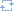Change directionMost popular convertion pairs of flow - mass

© converter.eu  |  API  |  feedback